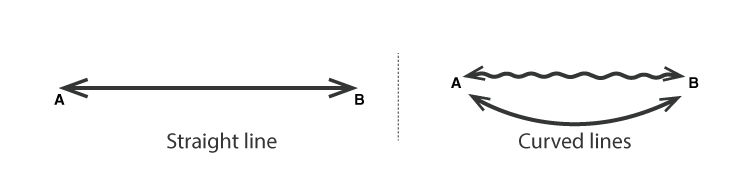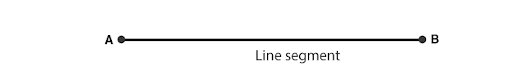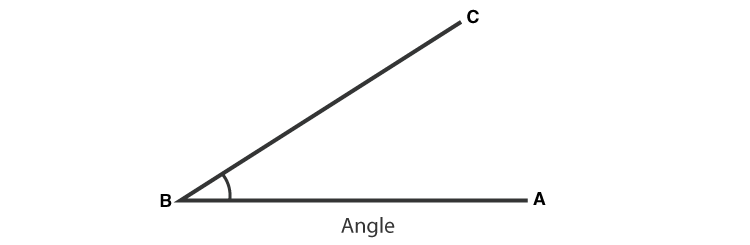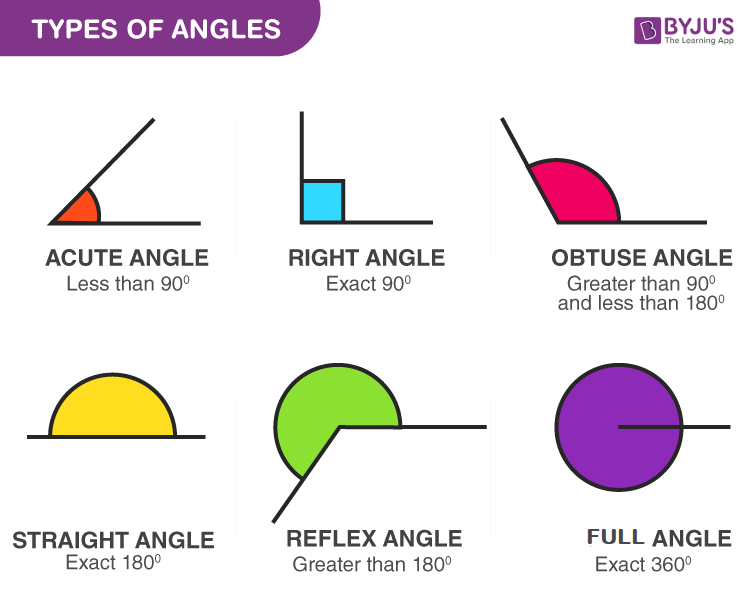# Lines And Angles Class 7

Lines and angles for Class 7 introduction start with introducing Geometry first. Geometry is derived from two Greek words, ‘Geo’, which means ‘Earth’ and ‘Metron’, which mean ‘Measurement’. So basically, Geometry deals with the measurement of different figures like Point, Line, Line Segment, Angles, Circles, Squares etc.

Geometry begins with a point which denotes a location and is represented by a dot(.). A point has no dimensions i.e. no length, no width and no height, but with the help of this point, we can draw lines and angles. Now, let us give basic knowledge of lines and angles which is introduced in Class 7 syllabus. Lines and angles class 7 provides knowledge about basic geometry. Here, we are going to discuss the various types of angle, its measure, parallel lines angle formed using lines, etc. In this article, we are going to discuss the introduction of lines and angles, and topics covered under this chapter.

## Lines and Angles Class 7 Topics

The topics and subtopics covered in lines and angles class 7 are:

• Introduction
• Related Angles
• Complementary angles
• Supplementary angles
• Linear pair
• Vertically opposite angles
• Pairs of Lines
• Intersecting lines
• Transversal
• Angles made by the transversal
• Transversal of Parallel Lines
• Checking for Parallel Lines

## Lines and Angles Class 7 Notes

Let us discuss the concepts covered in the class 7 lines and angles.

### Lines and Angles Introduction

To cover Lines and Angles Class 7 syllabus, we have generalized the topics here. Read the below topics one by one to understand the concepts of lines and angles.

Line:

A line is a straight figure which doesn’t have an endpoint and extends infinitely in opposite directions.Ray:

A ray is a straight line, which starts from a fixed point and moves in one direction.Line Segment

A portion of the line formed with two definite points is called a Line Segment. A line is a one-dimensional figure and has no thickness.Angle

When we join two line segments at a single point, an angle is formed, or we can say, an Angle is a combination of two line segments at a common endpoint. This common point is called Vertex of the angle and the two line segments are sides or arms of the angle formed.Types of Angles

There are basically 6 types of angles which are:

1. Acute Angle: If an angle is less than 90 degrees, then it is called an Acute angle
2. Obtuse Angle: If an angle is more than 90 degrees, then it is called Obtuse Angle
3. Right Angle: If an angle is exactly at 90 degrees, then it is called Right Angle.
4. Straight Angle: If an angle is exactly 180 degrees, then it is called Straight Angle.
5. Reflex Angle: If the angle is more than 180 degree but less than 270 degrees, it is denoted as a Reflex angle.
6. Full Angle: A 360-degree angle is called a Full angle.### Related Angles

Apart from this, the different angles are:

Complementary Angle: The sum of the measures of two angles is 90°

Supplementary Angle: The sum of the measures of two angles is 180°

Adjacent Angle: Adjacent angles have a common vertex and a common arm but no common interior points

Linear pair: A linear pair is a pair of adjacent angles whose non-common sides are opposite rays

Vertically Opposite Angles: when two lines intersect, the vertically opposite angles so formed are equal.

### Pairs of Lines

Intersecting lines: Two lines intersect if they have a point in common. This common point O is their point of intersection.

Transversal: A line that intersects two or more lines at distinct points is called a transversal.

Angles made by the transversal: There are different angles formed when the transversal cuts the lines. They are:

• Interior angles
• Exterior angles
• Pairs of Alternate interior angles
• Pairs of Alternate exterior angles
• Pairs of Corresponding angles
• Pairs of interior angles on the same side of the transversal

Transversal of Parallel Lines: We know that the parallel lines are the lines that do not meet anywhere. Transversals of parallel lines give rise to quite interesting results.

### Checking for Parallel Lines

If a transversal cuts two lines, such that, each pair of corresponding angles are equal in measure.

Similarly, if a transversal cuts two lines, then each pair of the alternate interior angles are equal.

Also, if the transversal cuts the lines, then each pair of interior angles on the same side of the transversal are supplementary.

In these cases, the lines have to be in the parallel condition.

Class 7 students should exercise problems on Lines and Angles to understand the concepts. Lines and Angles Class 7 ICSE, CBSE and Other State Boards covers almost the same topics as per mentioned above for lines and angles.

For questions based upon Lines and Angles topics, keep visiting BYJU’S. Also, register now to get access to various additional maths video lessons explained in an engaging and effective way.

Quiz on Lines and angles for class 7